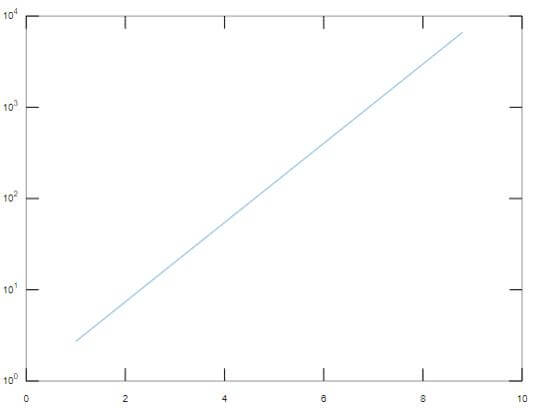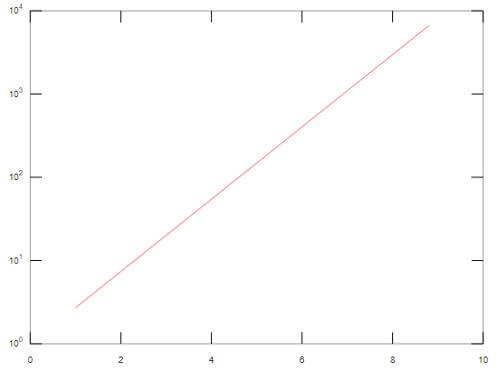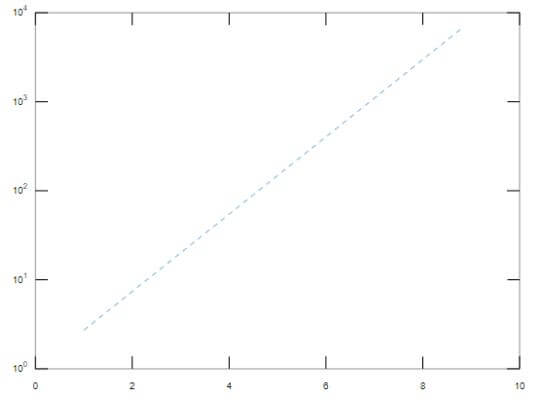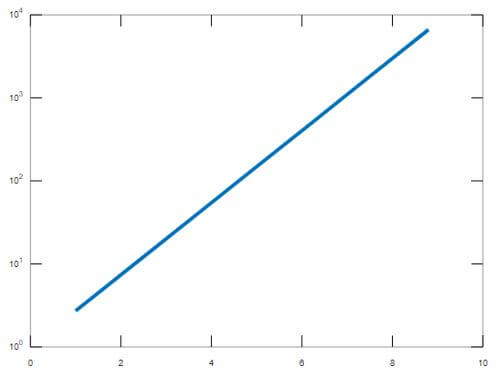# Semilogy Matlab | A Complete Guide to Semilogy Matlab

17## Introduction to Semilogy Matlab

Logarithmic plots are used to plot and implement the values that belong to a large scale, and the plot is nonlinear in nature. In Matlab, semilogy function is used to create the logarithmic plot with a base of 10, and there is a linear scale on the x-axis. These plots are used in various sectors like data science, artificial intelligence etc. It plots the graph if the vertical axis or y-axis contains real numbers.

### Working with Semilogy in Matlab with Syntax and Examples

In Matlab, semilogy is used for many applications. It is mainly used to plot the logarithmic scale for the values present in the y axis.

Given below are the syntaxes which are used in Matlab:

`S=semilogy(y)`

This syntax is used to plot the graph, which uses the logarithmic scale of base 10 for the values on the Y-axis, and it uses a linear scale for the values present on the x-axis. It is used to plot the value of the column of Y with respect to their index. If the value of Y has an imaginary component, then semilogy(y) will be equal to semilogy (real part of y, imaginary part of y).

`S=semilogy (x1, y1,… xn, yn)`

This syntax is used to plot the graph in the form of xn and yn pairs. If any of the value is a matrix, then it will plot the vector argument with respect to various values present in the rows and columns of a matrix along with the value of the dimension in a matrix in such a way that the length of it matches the respective length of the vector. The values of xn can be of type datetime, numeric, duration or categorical, while the values of yn should be always numeric in nature.

`S=semilogy (x1, y1, Line specification…)`

This syntax is used to modify the changes of the line, which can be Line Style, Marker Symbol, Marker Color of various lines plotted in the graph.

`S=semilogy (……, Property Name, Value of the respective property)`

This is used to modify the appearance of the plotted lines by changing the various properties. The property’s name can be given in the “Property Name” specification, while the value of the respective property can be assigned in the “Value “argument.

`S=semilogy (axes of the line...)`

This is used to plot the lines mentioned in the axes argument rather than the current axes.

`S=semilogy (…...)`

This is used to return a vector of line objects.

### Examples of Semilogy Matlab

Given below are the examples which depict the use of semilogy in Matlab.

#### Example #1

To create a plot with a logarithmic scale present on Y-axis and linear scale on the x-axis.

Code:

```a = 1:1.3:10; b = exp(a); s=semilogy(a,b)```

Output:In the above example, the scale on the y-axis is logarithmic in nature, while the scale on the x-axis is linear in nature.

Below properties that can be used in semilogy plot to change the appearance of the plotted lines like the line style, line width, line color etc.

Line color is one of the main property of the semilogy plot, which changes the color of the plotted line. The color can be any RGB triplet value, hexadecimal color code etc. RGB triplet value is the vector with three numeric values, and it describes the intensity levels of red, green and blue. The values of the intensity should be in the range of 0 to 1. The colors of the line can be red, green, blue, magenta, cyan, white, black, yellow.

Below example showing the code for changing the color of plotted line.

#### Example #2

To change the color of the plotted line to Red.

Code:

```a = 1:1.3:10; b = exp(a); s=semilogy(a,b,"Color","Red")```

Output:Line Style and Line width are the other two properties of the plotted lines, which are used to change the style and width of the plotted lines. Line style can be of different forms like a solid line, dashed lined, dotted line, dash-dotted line, where solid line is the default line. Similarly, Line width can be any positive numeric value.

The below example shows Line style and Line width property in Matlab with respect to semilogy.

#### Example #3

To show the property of Line Style using a dashed line.

Code:

```a = 1:1.3:10; b = exp(a); s=semilogy(a,b,"LineStyle","--")```

Output:#### Example #4

To show the property of Line Width using a set value.

Code:

```a = 1:1.3:10; b = exp(a); s=semilogy(a,b,"LineWidth",4)```

Output:Color Mode is one of the property which is used to control the color property of the plotted lines. It can take ‘auto’ and ‘manual ‘values. If the property’s value is set to auto, then Matlab is supposed to select a color from the Color property while if it is set to ‘manual’, then we can control the value of the Color property and change it manually. Similarly, there is another property which is known as Line Style Mode. It can take two values which are auto and manual. If the property’s value is set to auto, then Matlab is supposed to select a line style from the LineStyle property while if it is set to ‘manual’, then we can control the value of the LineStyle property and change it manually.

To sharpen the horizontal and vertical lines of the plot, there is another property called “AlignVertexCenters”. To remove the uneven appearance of the plot, we can use this property. If the value of the respective property is “OFF”, then it will not sharpen the horizontal and vertical lines, and the lines may appear uneven in the plot. If the respective property’s value is “ON”, it will sharpen the horizontal and vertical lines for a smooth appearance of the plotted lines.

### Conclusion

Various other properties are associated with semilogy plot in Matlab, like marker size, marker color etc., which controls the appearance of the plotted lines. Semilogy is an important function to learn in Matlab because of its vast applications.

### Recommended Articles

This is a guide to Semilogy Matlab. Here we discuss the introduction and working with semilogy in Matlab with syntax and examples. You may also have a look at the following articles to learn more –

The post Semilogy Matlab appeared first on EDUCBA.